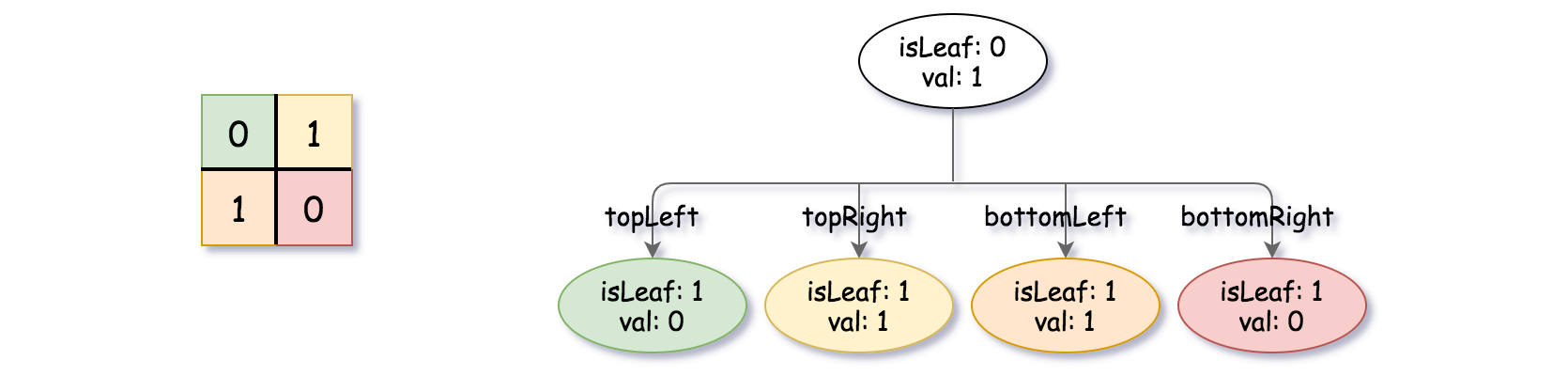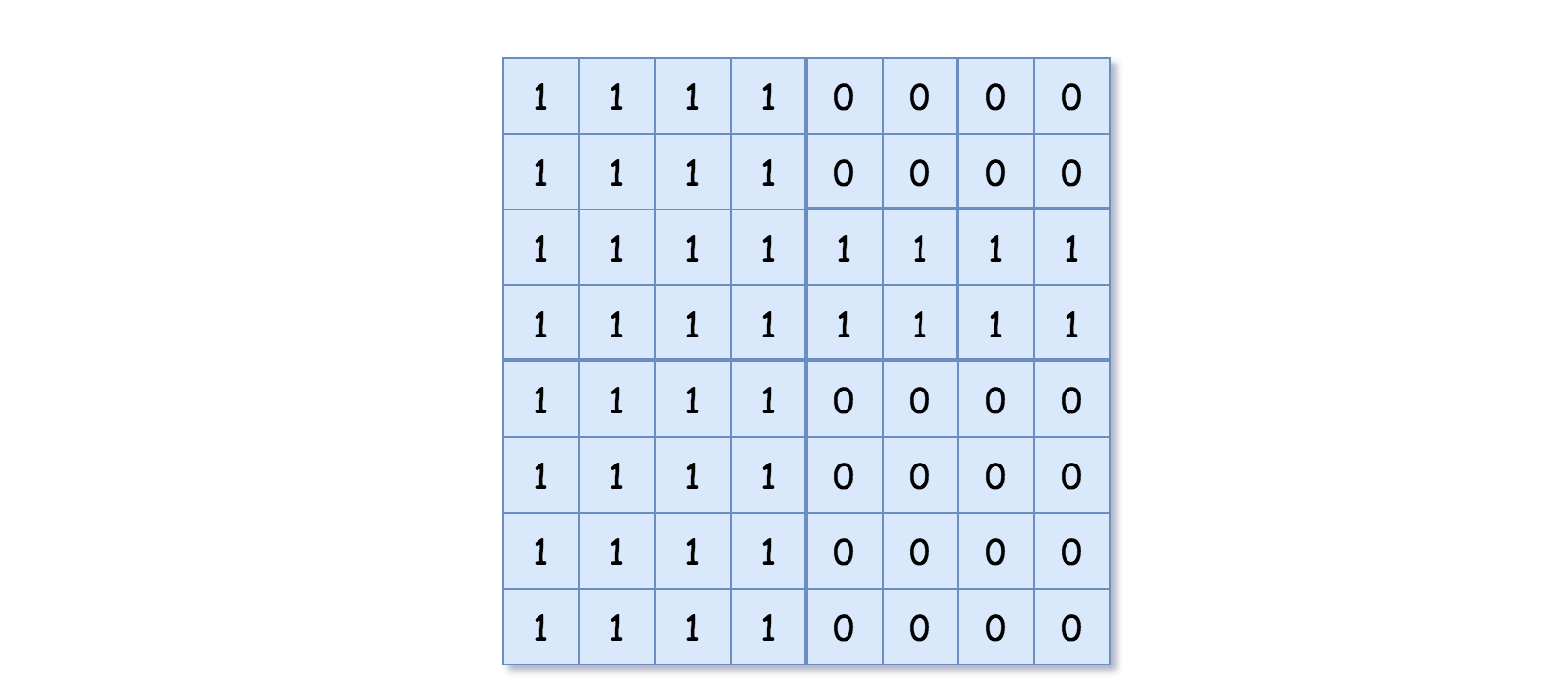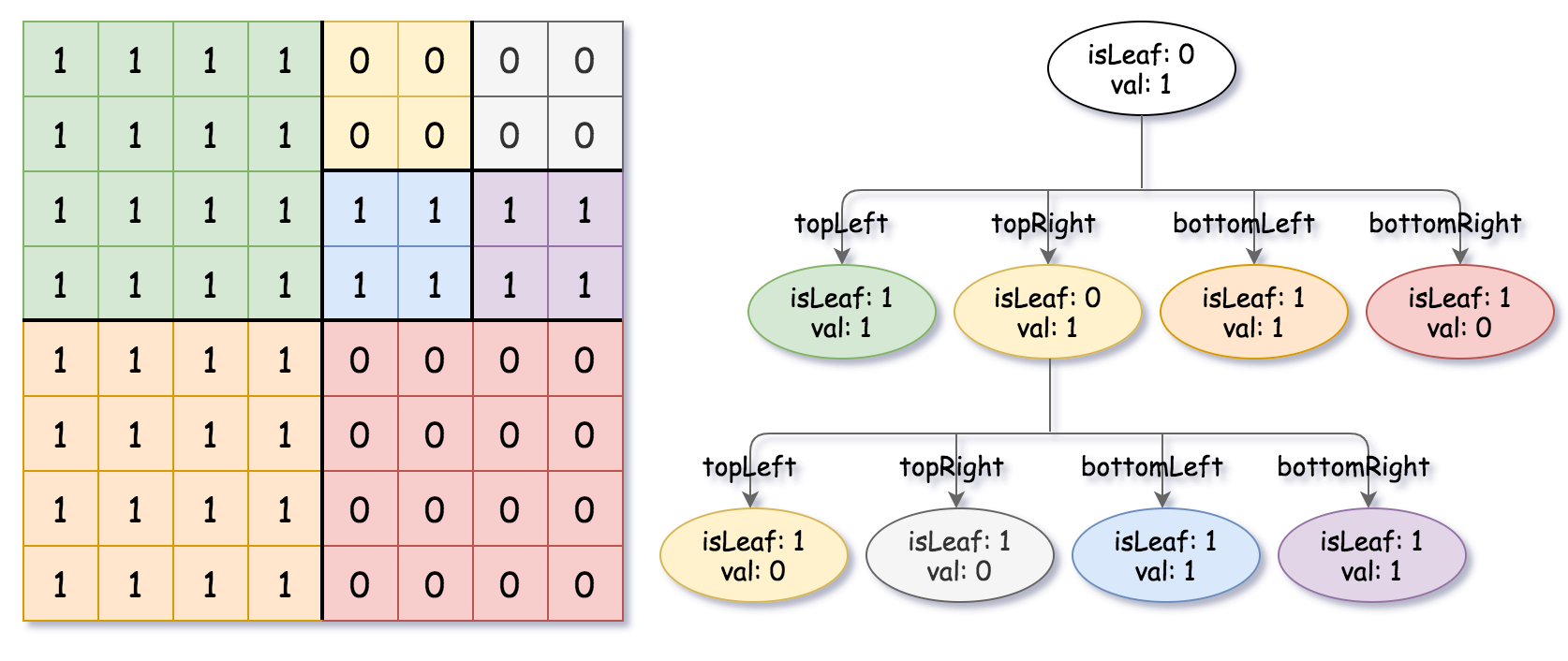## Algorithm

Problem Name: 427. Construct Quad Tree

Given a `n * n` matrix `grid` of `0's` and `1's` only. We want to represent the `grid` with a Quad-Tree.

Return the root of the Quad-Tree representing the `grid`.

Notice that you can assign the value of a node to True or False when `isLeaf` is False, and both are accepted in the answer.

A Quad-Tree is a tree data structure in which each internal node has exactly four children. Besides, each node has two attributes:

• `val`: True if the node represents a grid of 1's or False if the node represents a grid of 0's.
• `isLeaf`: True if the node is leaf node on the tree or False if the node has the four children.
```class Node {
public boolean val;
public boolean isLeaf;
public Node topLeft;
public Node topRight;
public Node bottomLeft;
public Node bottomRight;
}```

We can construct a Quad-Tree from a two-dimensional area using the following steps:

1. If the current grid has the same value (i.e all `1's` or all `0's`) set `isLeaf` True and set `val` to the value of the grid and set the four children to Null and stop.
2. If the current grid has different values, set `isLeaf` to False and set `val` to any value and divide the current grid into four sub-grids as shown in the photo.
3. Recurse for each of the children with the proper sub-grid.If you want to know more about the Quad-Tree, you can refer to the wiki.

The output represents the serialized format of a Quad-Tree using level order traversal, where `null` signifies a path terminator where no node exists below.

It is very similar to the serialization of the binary tree. The only difference is that the node is represented as a list `[isLeaf, val]`.

If the value of `isLeaf` or `val` is True we represent it as 1 in the list `[isLeaf, val]` and if the value of `isLeaf` or `val` is False we represent it as 0.

Example 1:```Input: grid = [[0,1],[1,0]]
Output: [[0,1],[1,0],[1,1],[1,1],[1,0]]
Explanation: The explanation of this example is shown below:
Notice that 0 represnts False and 1 represents True in the photo representing the Quad-Tree.```

Example 2:```Input: grid = [[1,1,1,1,0,0,0,0],[1,1,1,1,0,0,0,0],[1,1,1,1,1,1,1,1],[1,1,1,1,1,1,1,1],[1,1,1,1,0,0,0,0],[1,1,1,1,0,0,0,0],[1,1,1,1,0,0,0,0],[1,1,1,1,0,0,0,0]]
Output: [[0,1],[1,1],[0,1],[1,1],[1,0],null,null,null,null,[1,0],[1,0],[1,1],[1,1]]
Explanation: All values in the grid are not the same. We divide the grid into four sub-grids.
The topLeft, bottomLeft and bottomRight each has the same value.
The topRight have different values so we divide it into 4 sub-grids where each has the same value.
Explanation is shown in the photo below:```

Constraints:

• `n == grid.length == grid[i].length`
• `n == 2x` where `0 <= x <= 6`

## Code Examples

### #1 Code Example with Javascript Programming

```Code - Javascript Programming```

``````
class Solution {
public Node construct(int[][] grid) {
return helper(grid, 0, 0, grid.length);
}

private Node helper(int[][] grid, int x, int y, int len) {
if (len == 1) {
return new Node(grid[x][y] != 0, true, null, null, null, null);
}
Node result = new Node();
Node topLeft = helper(grid, x, y, len / 2);
Node topRight = helper(grid, x, y + len / 2, len / 2);
Node bottomLeft = helper(grid, x + len / 2, y, len / 2);
Node bottomRight = helper(grid, x + len / 2, y + len / 2, len / 2);
if (
topLeft.isLeaf &&
topRight.isLeaf &&
bottomLeft.isLeaf &&
bottomRight.isLeaf &&
topLeft.val == topRight.val &&
topRight.val == bottomLeft.val &&
bottomLeft.val == bottomRight.val
) {
result.isLeaf = true;
result.val = topLeft.val;
}
else {
result.topLeft = topLeft;
result.topRight = topRight;
result.bottomLeft = bottomLeft;
result.bottomRight = bottomRight;
}
return result;
}
}
``````
Copy The Code &

Input

cmd
grid = [[0,1],[1,0]]

Output

cmd
[[0,1],[1,0],[1,1],[1,1],[1,0]]

### #3 Code Example with Python Programming

```Code - Python Programming```

``````
class Solution:
def construct(self, grid):
def dfs(x, y, l):
if l == 1:
node = Node(grid[x][y] == 1, True, None, None, None, None)
else:
tLeft = dfs(x, y, l // 2)
tRight = dfs(x, y + l // 2, l // 2)
bLeft = dfs(x + l // 2, y, l// 2)
bRight = dfs(x + l // 2, y + l // 2, l // 2)
value = tLeft.val or tRight.val or bLeft.val or bRight.val
if tLeft.isLeaf and tRight.isLeaf and bLeft.isLeaf and bRight.isLeaf and tLeft.val == tRight.val == bLeft.val == bRight.val:
node = Node(value, True, None, None, None, None)
else:
node = Node(value, False, tLeft, tRight, bLeft, bRight)
return node
return grid and dfs(0, 0, len(grid)) or None
``````
Copy The Code &

Input

cmd
grid = [[1,1,1,1,0,0,0,0],[1,1,1,1,0,0,0,0],[1,1,1,1,1,1,1,1],[1,1,1,1,1,1,1,1],[1,1,1,1,0,0,0,0],[1,1,1,1,0,0,0,0],[1,1,1,1,0,0,0,0],[1,1,1,1,0,0,0,0]]

Output

cmd
[[0,1],[1,1],[0,1],[1,1],[1,0],null,null,null,null,[1,0],[1,0],[1,1],[1,1]]

### #4 Code Example with C# Programming

```Code - C# Programming```

``````
namespace LeetCode
{
{
public Node Construct(int[][] grid)
{
var N = grid.Length;
if (N == 0) return new Node(true, true);
if (N == 1) return new Node(grid == 1, true);

return DFS(grid, 0, N, 0, N);
}

private Node DFS(int[][] grid, int xMin, int xMax, int yMin, int yMax)
{
var same = true;
for (int i = xMin; i < xMax; i++)
{
for (int j = yMin; j < yMax; j++)
if (grid[i][j] != grid[xMin][yMin])
{
same = false;
break;
}
if (!same) break;
}

if (same) return new Node(grid[xMin][yMin] == 1, true);

var node = new Node();
var xMid = xMin + (xMax - xMin) / 2;
var yMid = yMin + (yMax - yMin) / 2;

node.topLeft = DFS(grid, xMin, xMid, yMin, yMid);
node.topRight = DFS(grid, xMin, xMid, yMid, yMax);
node.bottomLeft = DFS(grid, xMid, xMax, yMin, yMid);
node.bottomRight = DFS(grid, xMid, xMax, yMid, yMax);

return node;
}

public class Node
{
public bool val;
public bool isLeaf;
public Node topLeft;
public Node topRight;
public Node bottomLeft;
public Node bottomRight;

public Node()
{
val = false;
isLeaf = false;
topLeft = null;
topRight = null;
bottomLeft = null;
bottomRight = null;
}

public Node(bool _val, bool _isLeaf)
{
val = _val;
isLeaf = _isLeaf;
topLeft = null;
topRight = null;
bottomLeft = null;
bottomRight = null;
}

public Node(bool _val, bool _isLeaf, Node _topLeft, Node _topRight, Node _bottomLeft, Node _bottomRight)
{
val = _val;
isLeaf = _isLeaf;
topLeft = _topLeft;
topRight = _topRight;
bottomLeft = _bottomLeft;
bottomRight = _bottomRight;
}
}
}
}
``````
Copy The Code &

Input

cmd
grid = [[1,1,1,1,0,0,0,0],[1,1,1,1,0,0,0,0],[1,1,1,1,1,1,1,1],[1,1,1,1,1,1,1,1],[1,1,1,1,0,0,0,0],[1,1,1,1,0,0,0,0],[1,1,1,1,0,0,0,0],[1,1,1,1,0,0,0,0]]

Output

cmd
[[0,1],[1,1],[0,1],[1,1],[1,0],null,null,null,null,[1,0],[1,0],[1,1],[1,1]]